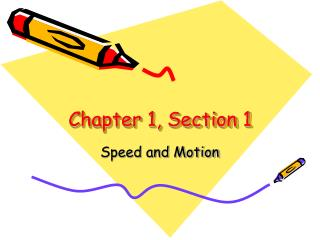DownloadDownload PresentationChapter 1, Section 1

# Chapter 1, Section 1

Download Presentation## Chapter 1, Section 1

- - - - - - - - - - - - - - - - - - - - - - - - - - - E N D - - - - - - - - - - - - - - - - - - - - - - - - - - -
##### Presentation Transcript

1. Chapter 1, Section 1 Speed and Motion

2. Recognizing Motion • What’s happening to the distance between the bus and the light posts as the bus is moving? • Which of the two objects is a reference point? So what is a reference point? • What reference points do you see when you walk to school or class?

3. Calculating Speed • What is the average speed limit on the freeway? • What are the two parts of a speed measurement? • Speed =

4. Calculating Speed Speed = What is the dog’s speed? 30m/15s = 2m/s time = 0 s distance = 0 m t = 15 s d = 30 m

5. Making a Speed Graph

6. Speed

7. Constant Speed • If a ship traveling in the ocean keeps the same speed for several hours, it is going at a _________ speed. • A horse ran 21 meters in 3 seconds, what was it’s speed? • 21 m ÷ 3 s = 7m/s

8. Average Speed • When a bicyclist is racing up and down mountains, his speed _______. • You can use the ________ speed to describe the motion of that bicyclist at every point during the race.

9. Average Speed • Average Speed = total distance ÷ total time • If a cyclist travels 32 km in the first 2 hours and 13 km in the last 1 hour, what was his average speed? • 32km + 13km = 45km • 2h + 1h = 3h • 45km ÷ 3h = 15km/h

10. Velocity • Velocity always includes speed and ___________. • Which is an example of velocity? • Wind at 30mi/hr heading east • Car traveling 65mi/hr

11. Exploring Motion Graphs • Is this graph showing a constant speed? • What is the total time & distance?

12. Exploring Motion Graphs • What happened during the 6th to 8th minutes? • What effect did that have on the average speed?

13. Exploring Motion Graphs • What is the difference between the jogger’s speed on the third day and the first day? • What is the total distance & time?

14. d s t Solving Speed Problems • Use the Speed Triangle • Figure out if you must find speed, time or distance from the problem. • Cover up the letter you are trying to find to see what you must do.

15. Speed, Time, Distance Practice Problems • Which has a greater speed, a hawk that travels 600m in 60s, or an eagle flying 60m in 5s? • How long would it take to travel 30 meters if you were running at a speed of 2 meters/second? • If your average speed in a car was 45 miles/hour, how far would you get if you drove for 4 hours?

16. Draw 3 Motion Graphs • Label the x and y-axis with time and distance. • The first graph should represent slow motion. • The second graph should represent a faster motion. • The third graph should represent an even faster motion.

17. Balloon Demonstration • Blow up the balloon and hold firmly shut. • Describe the motion of the balloon right now. • What could you do to make the balloon move? • Release the balloon and describe when the balloon changes its speed or direction.